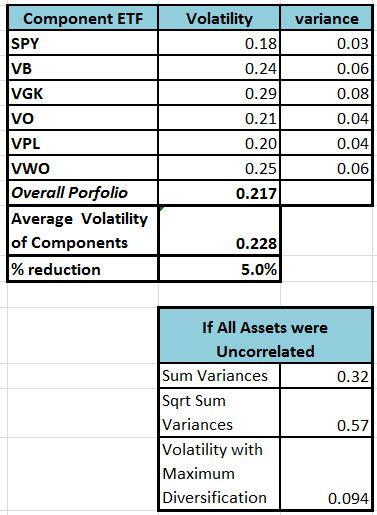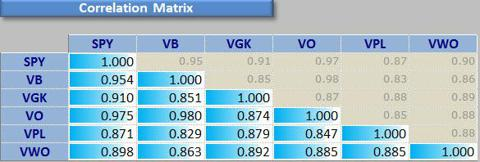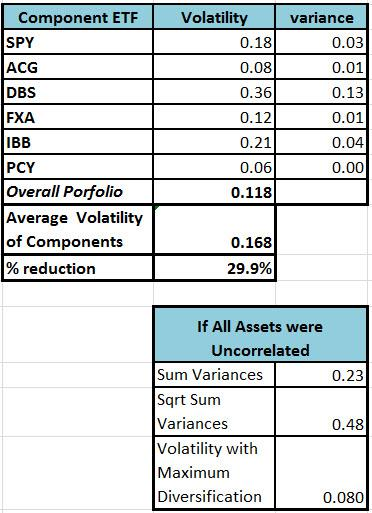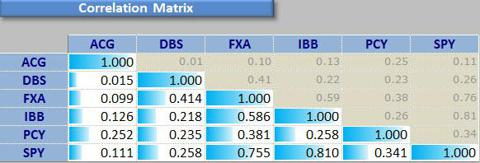# How To Assess Real-World Portfolio Diversification

|
Includes: ACG, DBS, FXA, IBB, PCY, SPY, VB, VGK, VO, VPL, VWO
by: John Dowdee

One of the central tenets of asset allocation is to select a diversified portfolio. The idea is intuitive; you do not keep all your eggs in one basket. Everyone talks about being diversified but there is little discussion on how you measure the degree of diversification. Surprisingly, when I began to research this topic, I found that there is no universally accepted formula for assessing diversification. In fact, the whole topic of diversification is typically clouded with hand waving and qualitative judgments.

In finance, diversification means reducing portfolio risk by investing in a variety of assets that do not move in lock-step with one another. There are many types of portfolio risk (interest rate risk, event risk, etc.) but for this analysis, I will define the portfolio risk to be synonymous with volatility. Diversification can then be characterized as the degree that you reduce the volatility of a portfolio by selecting assets.

To be "diversified," you want to choose assets such that when some assets are down, others are up. In mathematical terms, you want to select assets that are uncorrelated (or at least not highly correlated) with each other. In my definition of diversification, correlations will always be zero or positive. If the correlations are negative, I consider this to be "hedging" rather than diversifying.

I will discuss two methods of measuring diversification in a quantitative manner. Neither method is ideal but I have found that both are helpful in assessing if a portfolio is diversified.

It is well known that if you combine assets into a portfolio, the overall volatility (i.e. the standard deviation) is less than the average of volatilities associated with each of the components. So one measure of diversification is the percentage decrease achieved in the portfolio volatility as compared to the weighted average of the component volatilities. This is similar to the definition that has been offered in other Seeking Alpha articles such as this one.

As an example, consider an equally weighted portfolio (which I will call Portfolio 1) that consists of the following ETFs:

• SPY: SPDR S&P 500 Index
• VO: Vanguard Mid-Cap
• VB: Vanguard Small Cap
• VGK: Vanguard MSCI Europe
• VPL: Vanguard MSCI Pacific
• VWO: Vanguard FTSE Emerging Markets

On the surface this would appear to be a well-diversified portfolio with Large Caps, Mid-Caps, and Small Caps along with international stocks from Europe, the Pacific, and Emerging Markets.

To quantify the diversification, I plotted the annualized rate of return in excess of the risk free rate of return (called Excess Mu on the charts) against the historical volatility for each of the ETFs. The Smartfolio 3 program (smartfolio.com) was used to generate this plot based on three years of historical data. This data is shown in Figure 1.

Figure 1: Risk Reward for Portfolio 1

The volatility for each component is summarized in Figure 2 and the average of these volatilities is 22.8%. When these ETFs are combined into a portfolio, the resulting overall portfolio volatility is 21.7%, a reduction of 5%. This is not a large reduction in volatility, which suggests that this portfolio is not very diversified. This conclusion is validated by observing that the correlations among components are very high (see Figure 3).Figure 2: Portfolio 1 VolatilityFigure 3: Portfolio 1 Correlation Matrix

An alternative way to measure diversification is to compare your portfolio with the "maximum diversified" portfolio. I define a maximum diversification portfolio as one where all components are perfectly uncorrelated. Such a portfolio is not realizable in the real world but we can gain insight by comparing our portfolio with this idealized portfolio.

For a "maximum diversification" portfolio, all the correlation coefficients are zero so the portfolio variance is just the weighted sum of the individual variances. If the constituents are equally weighted, the portfolio standard deviation (i.e. the volatility) is calculated as: (square root of the sum of variance) divided by number of components.

For Portfolio 1, the volatility associated with maximum diversification is computed as 9.4% (see Figure 2). The overall volatility of Portfolio 1 was previously computed as 21.7%. This is long way from the ideal maximum diversification volatility. This is another indication that this is not a well-diversified portfolio.

In the current environment, finding a truly diversified portfolio is difficult since most assets have tended to move together during the bull market that began in 2009. This is not necessarily an issue and will likely not create any problems as long as the bulls stay strong. I understand that correlations change over time and an uncorrelated portfolio today may not be uncorrelated tomorrow. As with all things financial, you have to keep your eyes wide open and make adjustments to avoid risk. However, I am fearful that assets that rise together may also fall together, so I have continued to search for ETFs and CEFs that are not highly correlated.

As an example, let's consider Portfolio 2, another equally weighted portfolio consisting of the following ETFs and Closed End Funds (CEFs):

• SPY: SPDR S&P 500
• ACG: Alliance Bernstein Income Fund
• DBS: PowerShares DB Silver
• FXA: CurrencyShares Australian Dollar Trust
• IBB: iShares Nasdaq Biotechnology
• PCY: PowerShares Emerging Market Sovereign Debt

This portfolio goes further afield than the previous portfolio by including both commodity and currency assets. So the question is whether this portfolio is more diversified than Portfolio 1.

Figure 4 shows the Risk Reward plot for Portfolio 2, based again on 3 years of history. This portfolio delivers a rate of return similar to the S&P 500 but has substantially less volatility. The diversification statistics are summarized in Figure 5. The average volatility of the components is 16.8%. Combining these assets into a portfolio reduces the overall portfolio volatility to 11.8%, a reduction of almost 30%, much larger than for Portfolio 1. The volatility of the maximum diversification portfolio is 8%, which is not that far below the overall portfolio volatility. These measures are indicative of good diversification, which is verified by the correlation matrix shown in Figure 6. The correlations among SPY, IBB, and FXA are relatively high but the correlations among ACG, DBS, and PCY are relatively low. This illustrates that a few low correlations can be very beneficial in lowering the overall volatility of a portfolio.

Figure 4: Risk Reward for Portfolio 2Figure 5: Portfolio 2 VolatilityFigure 6: Portfolio 2 Correlation Matrix

To summarize, using quantitative measurements, Portfolio 2 is more diversified than Portfolio 1. Please note that I am not saying that Portfolio 1 is a "bad" portfolio. There are many ways to judge the suitability of a portfolio and diversification is only one of many factors to consider.

I also fully realize that my analysis of diversification is based on a set of assumptions that are only approximations to the real world. The data I presented is from the last three years, which was a tremendous bull market that is unlikely to repeat over the next three years. I welcome discussion on how diversification may or may not be a useful indicator for assessing portfolio risk going forward.

Disclosure: I am long DBS, FXA, PCY. I wrote this article myself, and it expresses my own opinions. I am not receiving compensation for it. I have no business relationship with any company whose stock is mentioned in this article.